Courses

# Stagnation and Sonic Properties Civil Engineering (CE) Notes | EduRev

## Civil Engineering (CE) : Stagnation and Sonic Properties Civil Engineering (CE) Notes | EduRev

The document Stagnation and Sonic Properties Civil Engineering (CE) Notes | EduRev is a part of the Civil Engineering (CE) Course Fluid Mechanics - Notes, Videos, MCQs & PPTs.
All you need of Civil Engineering (CE) at this link: Civil Engineering (CE)

Stagnation and Sonic Properties

• The stagnation properties at a point are defined as those which are to be obtained if the local flow were imagined to cease to zero velocity isentropically. As we will see in the later part of the text, stagnation values are useful reference conditions in a compressible flow.

Let us denote stagnation properties by subscript zero. Suppose the properties of a flow (such as T, p , ρ etc.) are known at a point, the stangation enthalpy is, thus, defined as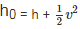where h is flow enthalpy and V is flow velocity.

• For a perfect gas , this yields,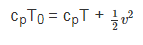(40.1)

Which defines the Stagnation Temperatur T

Now,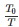can be expressed as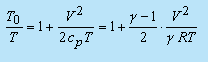Since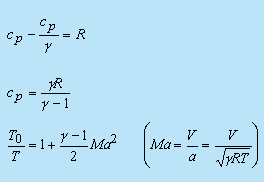(40.2)

If we know the local temperature (T) and Mach number (Ma) , we can find out the stagnation temperature T0 .

• Consequently, isentropic(adiabatic) relations can be used to obtain stagnation pressure and stagnation density as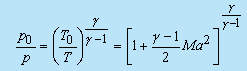(40.3)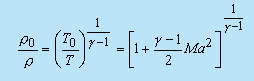(40.4)

Values of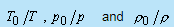as a function of Mach number can be generated using the above relationships and the tabulated results are known as Isentropic Table . Interested readers are suggested to refer the following books

1. J.Spruk, Fluid Mechanics, Springer, Heidelberg , NewYork, 1997
2. K.Muradidhar and G.Biswas, Advanced Engineering Fluid Mechanics, Second Edition, Narosa, 2005

Contd.

Note that in general the stagnation properties can vary throughout the flow field.

Let us consider some special cases :-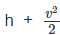from eqn 39.10) is constant throughout the flow. It follows that the h0, t0, α0 are constant throughout an adiabatic flow, even in the presence of friction.

Hence, all stagnation properties are constant along an isentropic flow. If such a flow starts from a large reservoir where the fluid is practically at rest, then the properties in the reservoir are equal to the stagnation properties everywhere in the flow Fig (40.1)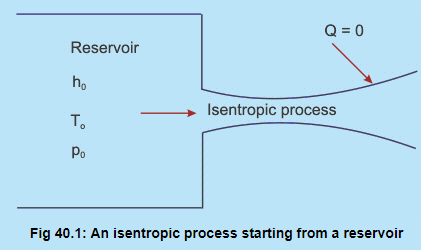Fig 40.1: An isentropic process starting from a reservoir

Case 2: Sonic Flow (Ma=1)

The sonic or critical properties are denoted by asterisks: p*ρ*, a*and T* . These properties are attained if the local fluid is imagined to expand or compress isentropically until it reaches Ma = 1.

Important-

The total enthalpy, hence T0 , is conserved as long as the process is adiabatic, irrespective of frictional effects.

From Eq. (40.1), we note that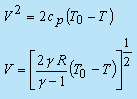(40.5a)

This gives the relationship between the fluid velocity V, and local temperature (T), in an adiabatic flow.

Putting T=0 we obtain maximum attainable velocity as,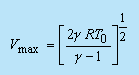(40.5b)

Considering the condition, when Mach number, Ma=1, for a compressible flow we can write from Eq. (40.2), (40.3) and (40.4),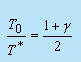(40.6a)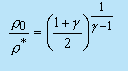(40.6b)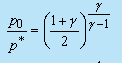(40.6c)

• For diatomic gases, like air   γ = 1.4 the numerical values are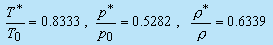• The fluid velocity and acoustic speed are equal at sonic condition and is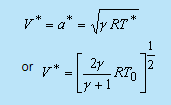( 40.7b)

We shall employ both stagnation conditions and critical conditions as reference conditions in a variety of one dimensional compressible flows.

Effect of Area Variation on Flow Properties in Isentropic Flow

In considering the effect of area variation on flow properties in isentropic flow, we shall determine the effect on the velocity V and the pressure p .

From Eq . (39.11), we can write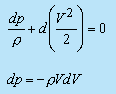Dividing by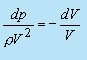(40.8)

A convenient differential form of the continuity equation can be obtained from Eq. (39.6) as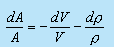Substituting from Eq. (40.8),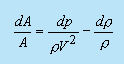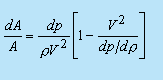(40.9)

Invoking the relation (39.3b) for isentropic process in Eq. (40.9), we get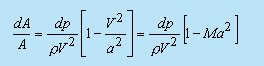(40.10)

• From Eq.(40.10), we see that for Ma<1 an area change causes a pressure change of the same sign, i.e. positive dA means positive dp for Ma<1 . For Ma>1 , an area change causes a pressure change of opposite sign.

• Again, substituting from Eq. (40.8) into Eq. (40.10), we obtain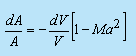(40.11)

From Eq. (40.11) we see that Ma<1 an area change causes a velocity change of opposite sign, i.e. positive dA means negative dV for Ma<1 . For Ma>1 an area change causes a velocity change of same sign.

These results can be summarized in fig 40.2. Equations (40.10) and (40.11) lead to the following important conclusions about compressible flows:

1. At subsonic speeds(Ma<1a decrease in area increases the speed of flow. A subsonic nozzle should have a convergent profile and a subsonic diffuser should possess a divergent profile. The flow behaviour in the regime of Ma<1 is therefore qualitatively the same as in incompressible flows.

2. In supersonic flows (Ma>1) the effect of area changes are different. According to Eq. (40.11), a supersonic nozzle must be built with an increasing area in the flow direction. A supersonic diffuser must be a converging channel. Divergent nozzles are used to produce supersonic flow in missiles and launch vehicles.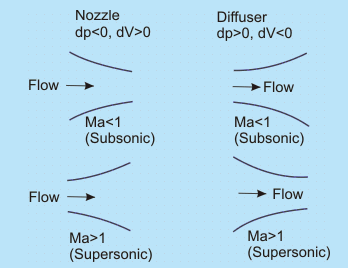Fig 40.2 Shapes of nozzles and diffusers in subsonic and supersonic regimes

onvergent - Divergent Nozzle

Suppose a nozzle is used to obtain a supersonic stream starting from low speeds at the inlet (Fig. 40.3). Then the Mach number should increase from Ma=0 near the inlet to Ma>1 at the exit. It is clear that the nozzle must converge in the subsonic portion and diverge in the supersonic portion. Such a nozzle is called a convergent-divergent nozzle. A convergent-divergent nozzle is also called a de laval nozzle, after Carl G.P. de Laval who first used such a configuration in his steam turbines in late nineteenth century.

From Fig. 40.3 it is clear that the Mach number must be unity at the throat, where the area is neither increasing nor decreasing. This is consistent with Eq. (40.11) which shows that dV can be nonzero at the throat only if Ma =1. It also follows that the sonic velocity can be achieved only at the throat of a nozzle or a diffuser.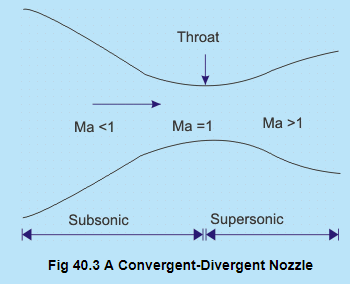The condition, however, does not restrict that Ma must necessarily be unity at the throat. According to Eq. (40.11), a situation is possible where Ma ≠ 1 at the throat if dV = 0 there. For an example, the flow in a convergent-divergent duct may be subsonic everywhere with Ma increasing in the convergent portion and decreasing in the divergent portion with Ma ≠ 1 at the throat (see Fig. 40.4).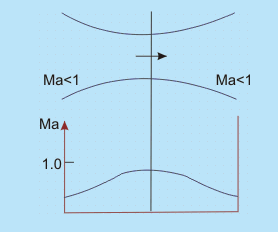Fig 40.4 Convergent-Divergent duct with Ma ≠ 1 at throat

The first part of the duct is acting as a nozzle, whereas the second part is acting as a diffuser. Alternatively, we may have a convergent divergent duct in which the flow is supersonic everywhere with Ma decreasing in the convergent part and increasing in the divergent part and again Ma ≠ 1 at the throat (see Fig. 40.5)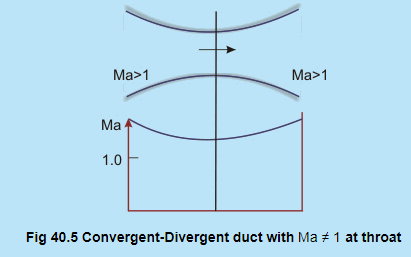Isentropic Flow in a Converging Nozzle

Consider the mass flow rate of an ideal gas through a converging nozzle. If the flow is isentropic, we can write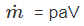where V  is flow velocity, A is area,  p is the density of the field.

This can equivalently be written as-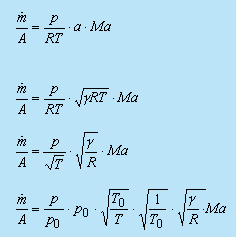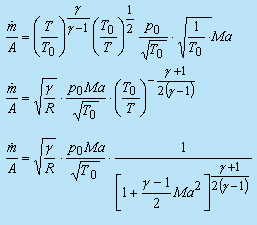(40.12)

In the expression (40.12),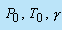and R are constant

The discharge per unit area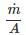is a function of Ma only. There exists a particular value of Ma for which it is maximum. Differentiating with respect to Ma and equating it to zero, we get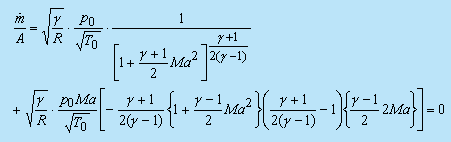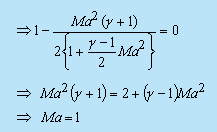Hence, discharge is maximum when Ma = 1.

• We know that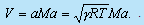By logarithmic differentiation, we get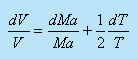(40.13)

We also know that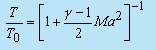By logarithmic differentiation, we get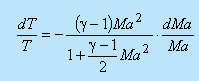(40.14)

From Eqs(40.13) and (40.14) , we get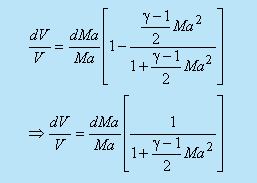(40.15)

From Eqs (40.11) and (40.15), we get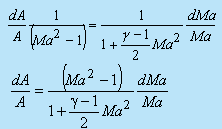(40.16)

By substituting Ma=1 in Eq. (40.16), we get dA = 0 or A = constant.

• Ma=1 can occur only at the throat and nowhere else, and this happens only when the discharge is maximum. When Ma = 1 , the discharge is maximum and the nozzle is said to be choked.

The properties at the throat are termed as critical properties which are already expressed through Eq. (40.6a), (40.6b) and (40.6c). By substituting Ma = 1 in Eq. (40.12), we get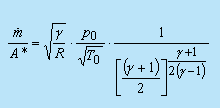(40.17)

(as we have earlier designated critical or sonic conditions by a superscript asterisk). Dividing Eq. (40.17) by Eq. (40.12) we obtain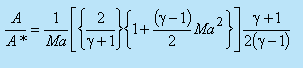(40.18)

From Eq. (40.18) we see that a choice of Ma gives a unique value of A/A* . The following figure shows variation of A / A * with Ma (Fig 40.6). Note that the curve is double valued; that is, for a given value of A/A* (other than unity), there are two possible values of Mach number. This signifies the fact that the supersonic nozzle is diverging.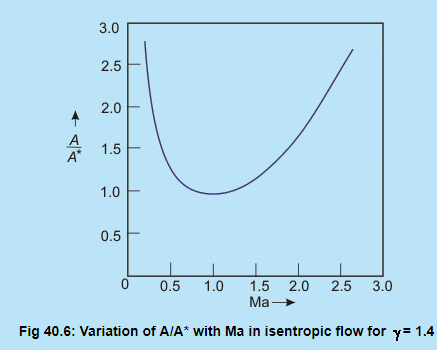The area ratio, as a function of Mach number, is also included in the Isentropic Table (see Spruk , Muralidhar and Biswas .

Pressure Distribution and Choking in a Converging Nozzle

• Consider a convergent nozzle as shown in Fig. 40.7(a). Figure 40.7(b) shows the pressure ratio p/ p0 along the length of the nozzle.

• The inlet conditions of the gas are at the stagnation state (p0, T0) which are constants. The pressure at the exit plane of the nozzle is denoted by PE and the back pressure is PB which can be varied by the adjustment of the valve. At the condition P0 = PE = Pthere shall be no flow through the nozzle.

• The pressure is P0 throughout, as shown by condition (i) in Fig. 40.7(b). As Pis gradually reduced, the flow rate shall increase. The pressure will decrease in the direction of flow as shown by condition (ii) in Fig. 40.7(b). The exit plane pressure PE shall remain equal to PB so long as the maximum discharge condition is not reached. Condition (iii) in Fig. 40.7(b) illustrates the pressure distribution in the maximum discharge situation.

• WhenAttains its maximum value, given by substituting Ma = 1 in Eq. (40.12), PE is equal to p* . Since the nozzle does not have a diverging section, further reduction in back pressure PB will not accelerate the flow to supersonic condition. As a result, the exit pressure PE shall continue to remain at p * even though PBis lowered further.

• The convergent-nozzle discharge against the variation of back pressure is shown in Fig. 40.8. We are aware, that the maximum value of (m/A) at Ma = 1 is stated as the choked flow. With a given nozzle, the flow rate cannot be increased further. Thus neither the nozzle exit pressure, nor the mass flow rate are affected by lowering PB below p *.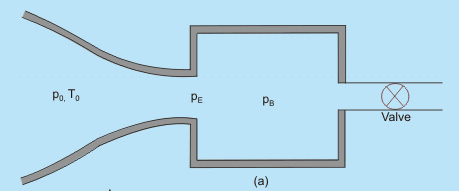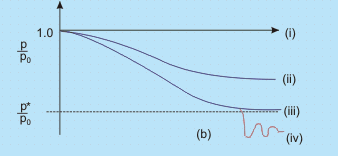Fig 40.7 (a) Compressible flow through a converging nozzle
(b) Pressure distribution along a converging nozzle for different values of back pressure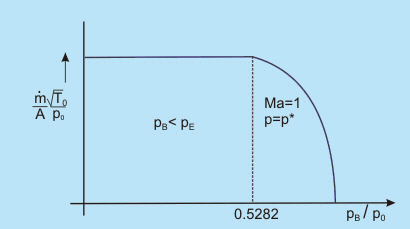Fig. 40.8 Mass flow rate and the variation of back pressure in a converging nozzle

However for PB less than p *, the flow leaving the nozzle has to expand to match the lower back pressure as shown by condition (iv) in Fig. 40.7(b). This expansion process is three-dimensional and the pressure distribution cannot be predicted by one-dimensional theory. Experiments reveal that a series of shocks form in the exit stream, resulting in an increase in entropy.

Isentropic Flow in a Converging-Diverging Nozzle

• Consider the flow in a convergent-divergent nozzle (Fig. 40.9). The upstream stagnation conditions are assumed constant; the pressure in the exit plane of the nozzle is denoted by PE ; the nozzle discharges to the back pressure, PB

• With the valve initially closed, there is no flow through the nozzle; the pressure is constant at P0. Opening the valve slightly produces the pressure distribution shown by curve (i). Completely subsonic flow is discerned.

• Then Pis lowered in such a way that sonic condition is reached at the throat (ii). The flow rate becomes maximum for a given nozzle and the stagnation conditions.

• On further reduction of the back pressure, the flow upstream of the throat does not respond. However, if the back pressure is reduced further (cases (iii) and (iv)), the flow initially becomes supersonic in the diverging section, but then adjusts to the back pressure by means of a normal shock standing inside the nozzle. In such cases, the position of the shock moves downstream as PB is decreased, and for curve (iv) the normal shock stands right at the exit plane.

• The flow in the entire divergent portion up to the exit plane is now supersonic. When the back pressure is reduced even further (v), there is no normal shock anywhere within the nozzle, and the jet pressure adjusts to Pby means of oblique shock waves outside the exit plane. A converging diverging nozzle is generally intended to produce supersonic flow near the exit plane.

• If the back pressure is set at (vi), the flow will be isentropic throughout the nozzle, and supersonic at nozzle exit. Nozzles operating at PB (corresponding to curve (vi) in Fig. 40.8) are said to be at design conditions. Rocket-propelled vehicles use converging-diverging nozzles to accelerate the exhaust gases to the maximum possible velocity to produce high thrust.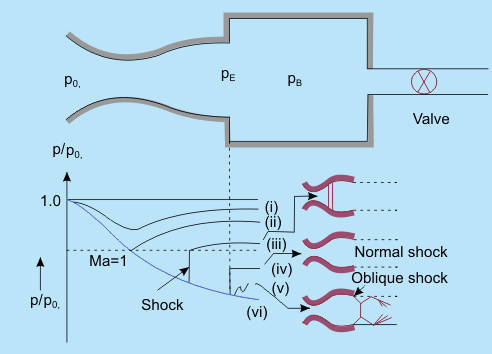Fig 40.9: Pressure Distribution along a Converging-Diverging Nozzle for different values of back pressure PB

Offer running on EduRev: Apply code STAYHOME200 to get INR 200 off on our premium plan EduRev Infinity!

,

,

,

,

,

,

,

,

,

,

,

,

,

,

,

,

,

,

,

,

,

;